# Translating Chemical Reactions to Equations Chemistry Reg GT

• Slides: 8Translating Chemical Reactions to Equations Chemistry Reg & GT 2/5/14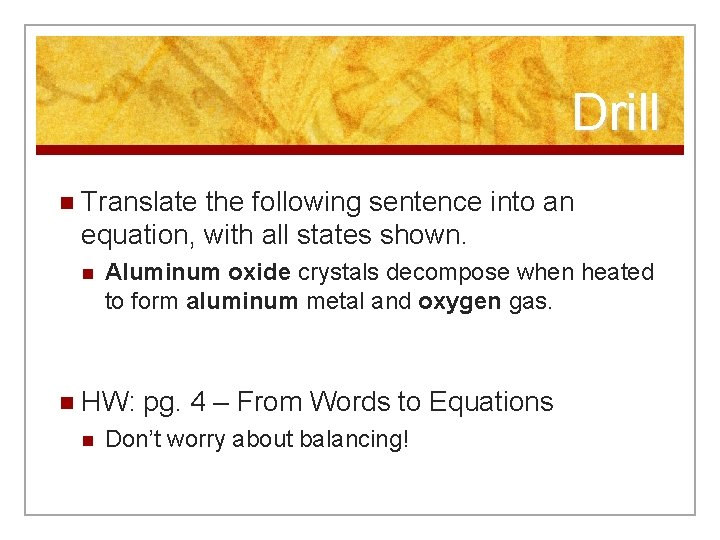Drill n Translate the following sentence into an equation, with all states shown. n Aluminum oxide crystals decompose when heated to form aluminum metal and oxygen gas. n HW: n pg. 4 – From Words to Equations Don’t worry about balancing!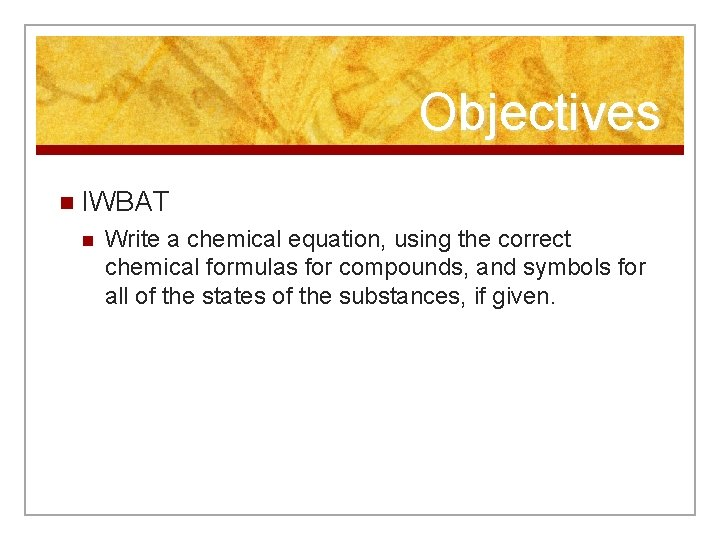Objectives n IWBAT n Write a chemical equation, using the correct chemical formulas for compounds, and symbols for all of the states of the substances, if given.Writing Equations n Example: n n Step 1: n n n diatomic elements? Put text into word equation: HIFNOBr. Cl Sodium + water hydrogen + sodium hydroxide Step 2: n n n Do you remember Sodium metal is added to water. Hydrogen gas the bubbles off, and sodium hydroxide is left behind, in solution. Br. O FINCl. H Translate words in word equation to formulas Na + H 2 O H 2 + Na. OH Step 3: n n Hockey Stick & Puck Add symbols to tell states of reactants and products. Na(s) + H 2 O(l) H 2(g) + Na. OH(aq)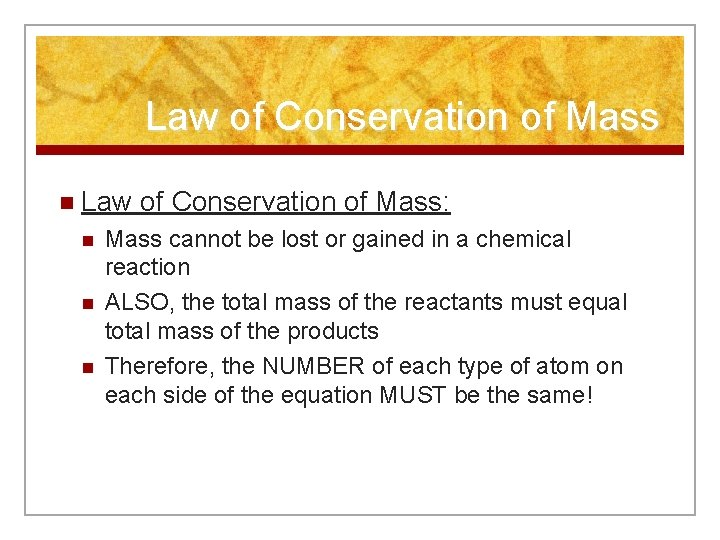Law of Conservation of Mass n Law n n n of Conservation of Mass: Mass cannot be lost or gained in a chemical reaction ALSO, the total mass of the reactants must equal total mass of the products Therefore, the NUMBER of each type of atom on each side of the equation MUST be the same!Practice n Go back to pg. 1 n Let’s review Part 1 & 2 n Work on Part 3 n n n Write the word equation on the first line Write an unbalanced equation on the second line Skip step c) for now n HW Review – pg. 3 questions on boards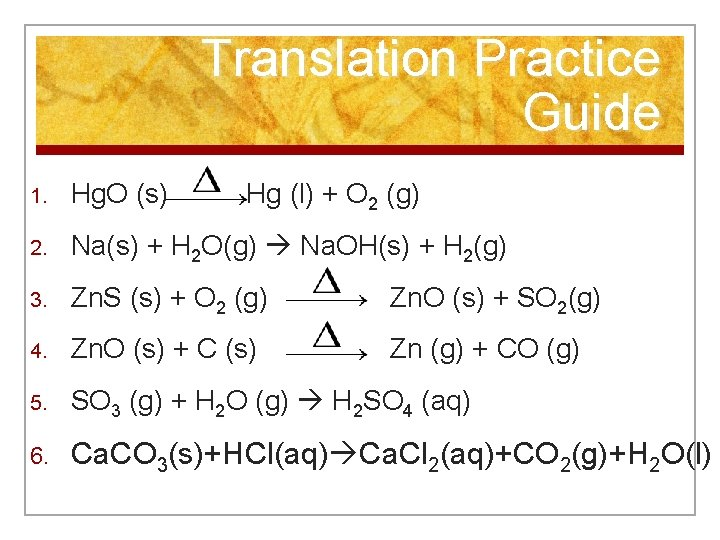Translation Practice Guide 1. Hg. O (s) Hg (l) + O 2 (g) 2. Na(s) + H 2 O(g) Na. OH(s) + H 2(g) 3. Zn. S (s) + O 2 (g) Zn. O (s) + SO 2(g) 4. Zn. O (s) + C (s) Zn (g) + CO (g) 5. SO 3 (g) + H 2 O (g) H 2 SO 4 (aq) 6. Ca. CO 3(s)+HCl(aq) Ca. Cl 2(aq)+CO 2(g)+H 2 O(l)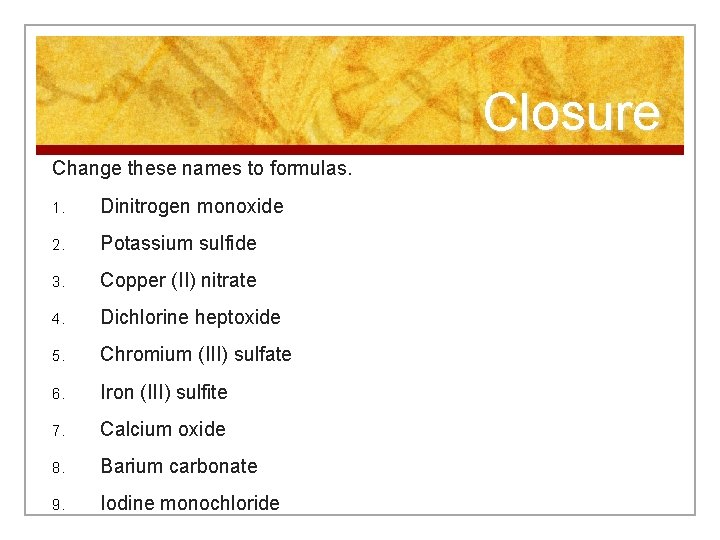Closure Change these names to formulas. 1. Dinitrogen monoxide 2. Potassium sulfide 3. Copper (II) nitrate 4. Dichlorine heptoxide 5. Chromium (III) sulfate 6. Iron (III) sulfite 7. Calcium oxide 8. Barium carbonate 9. Iodine monochloride